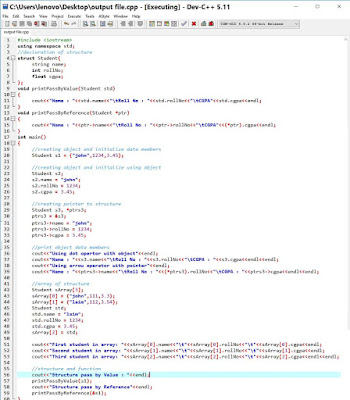## Use of Structures in C++

An array is a collection of variables of the same data types as to store roll numbers of 10 students, better to use an array of size 10 instead of using 10 variables.

Suppose we have to store details of 10 students as student name, roll number, and CGPA where the data type of each is different. For this we use structure. So we can say that.

Structure in C/C++ is a collection of variables of the same or different data types under a single name

## Structure declaration in C/C++

A structure can be declared either inside the main function or before the main function. Syntax of struct declaration is as below

struct structure-name{

data members

};

Below is the code for declaring a structure named Student.

struct Student{
string name;
int rollNo;
float cgpa;
};

Student structure contains three variables i.e name, rollNo and cpga. These variables are called data members.

The structure is also a data type but unlike int, float, char , etc which are primitive data types, the structure is a user-defined data type.

## Create an object of the structure

We can create an object of structure Student as below

Student s1;

## Pointer to structure

We can declare a pointer to structure like

Student s1,*ptrs1;

Here ptrs1 is a pointer to the structure

We can access data members of the structure using object or pointer to structure.

## Array of structure

We can declare array of structure like

Student sArr;

Here sArray is array of structures of 5 students

We can fill this array with objects of students as below

sArr={"laim",12,3.5};
sArr={"john",43,3.7};

## Or

Student s1;
s1.name = "laim";
s1.rollNo = 1234;
s1.cgpa = 3.45;
sArray[0= s1;

## We can display data members of objects in array like this

cout<<sArray.name;
cout<<sArray.rollNo;
cout<<sArray.name;
cout<<sArray.rollNo;
cout<<sArray.name;
cout<<sArray.rollNo;

## Structure initialization

Structure initialization means initializing the data members of a structure. There are three methods to initialize data members of a structure.

## First Method: At the time of object creation

By this method, an object is created and data members are initialized in a single line as below

Student s1={
"john",
234,
3.57
};

First data member name is initialized by  "ali"

Second data member rollNo is initialized by 231

Third data member cgpa is initialized by 3.2

## Second Method: Using dot (.) operator with object

Using this method,  first structure object is created as below

Student s1;

Then using this object s1, data members are initialized as below

s1.name = "john";
s1.rollNo = 1234;
s1.cgpa = 3.45;

## Third Method: Using arrow operator (->) with pointer

In this method, pointer to structure is created as below

Student s1, *ptrs1;
ptrs1 = &s1;

Then with help of arrow operator with pointer, data members are initialized as below

ptrs1->name = "john";
ptrs1->rollNo = 1234;
ptrs1->cgpa = 3.45;

We can use dot operator with a pointer to structure. For this, dereferencing the pointer is necessary as below

(*ptrs1).name = "john";
(*ptrs1).rollNo = 1234;
(*ptrs1).cgpa = 3.45;

## Structure and function

We can pass a structure as an argument to a function. A structure can be passed either by value or by reference.

## Structure pass by value

void print(Student s)
{
cout<<s.name;
}
Student s1={
"john",
23,
3.34
};
print(s1);

Output: john

## Structure pass by reference

void print(Student *ptr)
{
cout<<ptr->name;
}
Student s1={
"john",
23,
3.34
};
print(&s1);

Output: john

Below is a complete C++ program to cover all concepts of structureC++ Structure
Output

1.